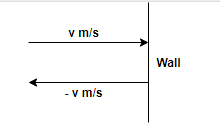# Problem: The average speed of a nitrogen molecule in air is about 6.70×10 2 m/s, and its mass is 4.68×10−26 kg.(a) If it takes 3.00×10−13 s for a nitrogen molecule to hit a wall and rebound with the same speed but moving in the opposite direction, what is the average acceleration of the molecule during this time interval?(b) What average force does the molecule exert on the wall?

###### FREE Expert Solution

We're asked for the acceleration and force involved in reversing a particle's velocity.

This problem involves forces in kinematics with horizontal acceleration.

Whenever we are given such a problem we apply the following steps.

1. Draw a free body diagram (FBD) (usually—this problem doesn't really need it, since only one force is acting.)
2. Apply kinematics equations and F=ma
3. Solve for the target variable

For part 2, we'll need to use the kinematic equations,

Step 1: Draw a free body diagram (FBD)

We'll take a coordinate system where the positive x-axis points to the right.

Therefore, velocity before the collision with the wall is positive and velocity after the collision (rebounce) with the wall is negative.Step 2: Apply kinematics equations and F=ma

99% (282 ratings)###### Problem Details

The average speed of a nitrogen molecule in air is about 6.70×10 2 m/s, and its mass is 4.68×10−26 kg.
(a) If it takes 3.00×10−13 s for a nitrogen molecule to hit a wall and rebound with the same speed but moving in the opposite direction, what is the average acceleration of the molecule during this time interval?
(b) What average force does the molecule exert on the wall?